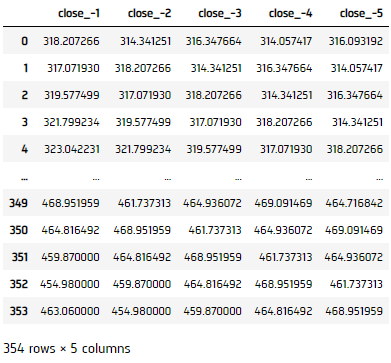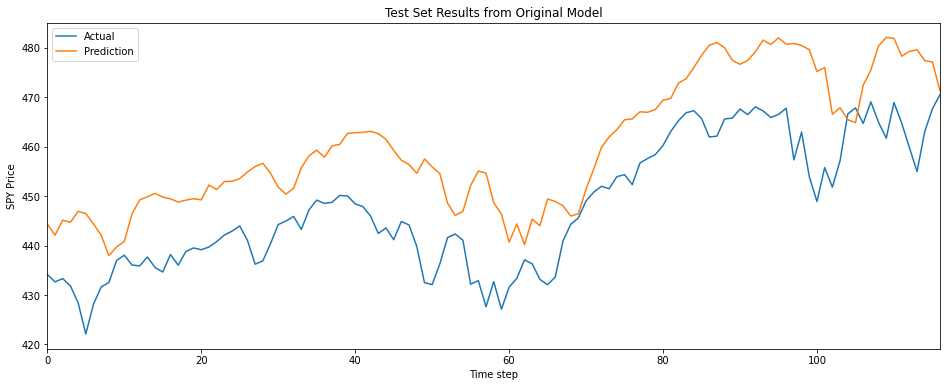# Machine Learning

## TensorFlow

### Introduction

This page explains how to build, train, test, and store Tensorflow models.

### Import Libraries

Import the tensorflow, sklearn, json5 and google.protobuf libraries.

import tensorflow as tf
from sklearn.model_selection import train_test_split
import json5
from google.protobuf import json_format

You need the sklearn library to prepare the data and the json5 and google.protobuf libraries to save models.

### Get Historical Data

Get some historical market data to train and test the model. For example, to get data for the SPY ETF during 2020 and 2021, run:

qb = QuantBook()
history = qb.History(symbol, datetime(2020, 1, 1), datetime(2022, 1, 1)).loc[symbol]

### Prepare Data

You need some historical data to prepare the data for the model. If you have historical data, manipulate it to train and test the model. In this example, use the following features and labels:

Data CategoryDescription
FeaturesThe last 5 closing prices
LabelsThe following day's closing price

Follow these steps to prepare the data:

1. Loop through the DataFrame of historical prices and collect the features.
2. lookback = 5
lookback_series = []
for i in range(1, lookback + 1):
df = history['close'].shift(i)[lookback:-1]
df.name = f"close_-{i}"
lookback_series.append(df)
X = pd.concat(lookback_series, axis=1).reset_index(drop=True)

The following image shows the format of the features DataFrame:3. Select the close column and then call the shift method to collect the labels.
4. Y = history['close'].shift(-1)
5. Drop the first 5 features and then call the reset_index method.
6. Y = Y[lookback:-1].reset_index(drop=True)

This method aligns the history of the features and labels.

7. Call the train_text_split method with the datasets and a split size.
8. For example, to use the last third of data to test the model, run:

X_train, X_test, y_train, y_test = train_test_split(X, Y, test_size=0.33, shuffle=False)

### Train Models

You need to prepare the historical data for training before you train the model. If you have prepared the data, build and train the model. In this example, build a neural network model that predicts the future price of the SPY.

#### Build the Model

Follow these steps to build the model:

1. Call the reset_default_graph method.
2. tf.reset_default_graph()

This method clears the default graph stack and resets the global default graph.

3. Call the Session constructor.
4. sess = tf.Session()
5. Declare the number of factors and then create placeholders for the input and output layers.
6. num_factors = X_test.shape
X = tf.placeholder(dtype=tf.float32, shape=[None, num_factors], name='X')
Y = tf.placeholder(dtype=tf.float32, shape=[None])
7. Set up the weights and bias initializers for each layer.
8. weight_initializer = tf.variance_scaling_initializer(mode="fan_avg", distribution="uniform", scale=1)
bias_initializer = tf.zeros_initializer()
9. Create hidden layers that use the Relu activator.
10. num_neurons_1 = 32
num_neurons_2 = 16
num_neurons_3 = 8

W_hidden_1 = tf.Variable(weight_initializer([num_factors, num_neurons_1]))
bias_hidden_1 = tf.Variable(bias_initializer([num_neurons_1]))

W_hidden_2 = tf.Variable(weight_initializer([num_neurons_1, num_neurons_2]))
bias_hidden_2 = tf.Variable(bias_initializer([num_neurons_2]))

W_hidden_3 = tf.Variable(weight_initializer([num_neurons_2, num_neurons_3]))
bias_hidden_3 = tf.Variable(bias_initializer([num_neurons_3]))
hidden_3 = tf.nn.relu(tf.add(tf.matmul(hidden_2, W_hidden_3), bias_hidden_3))
11. Create the output layer and give it a name.
12. W_out = tf.Variable(weight_initializer([num_neurons_3, 1]))
bias_out = tf.Variable(bias_initializer())
output = tf.transpose(tf.add(tf.matmul(hidden_3, W_out), bias_out), name='outer')

This snippet creates a 1-node output for both weight and bias. You must name the output layer so you can access it after you load and save the model.

13. Set up the loss function and optimizers for gradient descent optimization and backpropagation.
14. loss = tf.reduce_mean(tf.squared_difference(output, Y))
optimizer = tf.train.AdamOptimizer().minimize(loss)

Use mean-square error as the loss function because the close price is a continuous data and use Adam as the optimizer because of its adaptive step size.

15. Set the batch size and number of epochs to bootstrap the training process.
16. batch_size = len(y_train) // 10
epochs = 20

#### Train the Model

Follow these steps to train the model:

1. Call the run method with the result from the global_variables_initializer method.
2. sess.run(tf.global_variables_initializer())
3. Loop through the number of epochs, select a subset of the training data, and then call the run method with the subset of data.
4. for _ in range(epochs):
for i in range(0, len(y_train) // batch_size):
start = i * batch_size
batch_x = X_train[start:start + batch_size]
batch_y = y_train[start:start + batch_size]
sess.run(optimizer, feed_dict={X: batch_x, Y: batch_y})

### Test Models

To test the model, we'll setup a method to plot test set predictions ontop of the SPY price.

def test_model(sess, output, title, X):
prediction = sess.run(output, feed_dict={X: X_test})
prediction = prediction.reshape(prediction.shape, 1)

y_test.reset_index(drop=True).plot(figsize=(16, 6), label="Actual")
plt.plot(prediction, label="Prediction")
plt.title(title)
plt.xlabel("Time step")
plt.ylabel("SPY Price")
plt.legend()
plt.show()

test_model(sess, output, "Test Set Results from Original Model", X)### Store Models

You can save and load TensorFlow models using the Object Store.

#### Save Models

Follow these steps to save models in the Object Store:

1. Export the TensorFlow graph as a JSON object.
2. graph_definition = tf.compat.v1.train.export_meta_graph()
json_graph = json_format.MessageToJson(graph_definition)
3. Export the TensorFlow weights as a JSON object.
4. # Define a function to get the weights from the tensorflow session
def get_json_weights(sess):
weights = sess.run(tf.compat.v1.trainable_variables())
weights = [w.tolist() for w in weights]
weights_list = json5.dumps(weights)
return weights_list

json_weights = get_json_weights(sess)
sess.close()    # Close the session opened by the get_json_weights function
5. Save the graph and weights to the Object Store.
6. qb.ObjectStore.Save('graph', json_graph)
qb.ObjectStore.Save('weights', json_weights)

You must save a model into the Object Store before you can load it from the Object Store. If you saved a model, follow these steps to load it:

1. Read the model graph and weights from the Object Store.
2. json_graph = qb.ObjectStore.Read('graph')
json_weights = qb.ObjectStore.Read('weights')
3. Restore the TensorFlow graph from the JSON object.
4. tf.reset_default_graph()
graph_definition = json_format.Parse(json_graph, tf.compat.v1.MetaGraphDef())
sess = tf.Session()
tf.compat.v1.train.import_meta_graph(graph_definition)
5. Select the input and output tensors.
6. X = tf.compat.v1.get_default_graph().get_tensor_by_name('X:0')
output = tf.compat.v1.get_default_graph().get_tensor_by_name('outer:0')
7. Restore the model weights from the JSON object.
8. weights = [np.asarray(x) for x in json5.loads(json_weights)]
assign_ops = []
feed_dict = {}
vs = tf.compat.v1.trainable_variables()
zipped_values = zip(vs, weights)
for var, value in zipped_values:
value = np.asarray(value)
assign_placeholder = tf.placeholder(var.dtype, shape=value.shape)
assign_op = var.assign(assign_placeholder)
assign_ops.append(assign_op)
feed_dict[assign_placeholder] = value
sess.run(assign_ops, feed_dict=feed_dict)

You can also see our Videos. You can also get in touch with us via Discord.